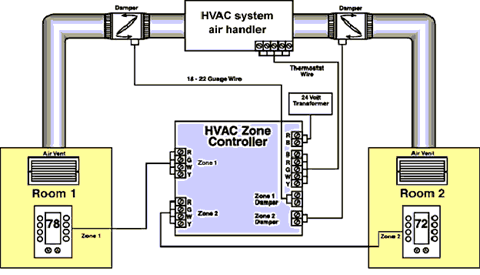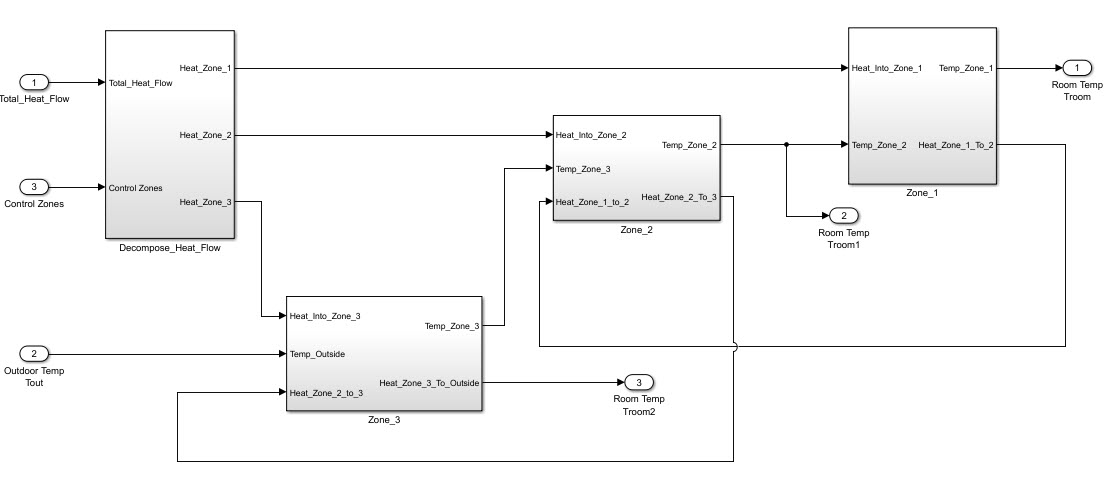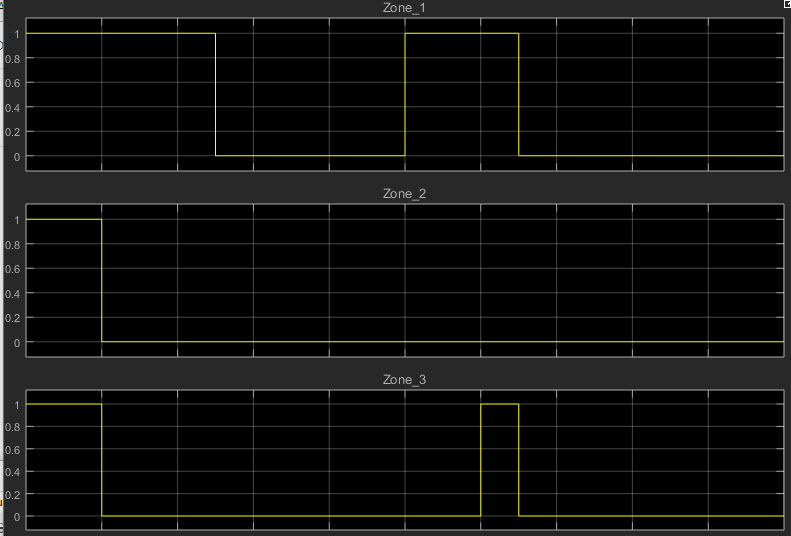## The most challanging control problem: Home heating for multi-floor homes

Hot air rises; cold air settles.  This is a fundamental law of nature and yet most multi-level homes are not set up to handle this challenge (including mine).  Having recently tried a “smart thermostat” and been very disappointed in it’s performance I have started to conceptually design my own home solution.

## Trial 1:  Zone alone

My first trial implementation was a simple manual “zone control” system; e.g. in the winter, the top floors are shut off so the heat rises, in summer the bottom is shut off.This approach worked somewhat but since I have a three story house and the thermostat is on the middle floor I always had a temperature gradient that was greater then I liked.

## Trial 2: Internet of things (IOT) and active zone control

We are now entering into the thought experiment part of the post.In the diagram above S1 ~ S3 are simple temperature sensors that would connect over wifi to the master control (hence the IOT part of this project).  The “Master Controller” would have open and close the ducts for each zone and control the Fan and heating and cooling elements.

## So far so standard…

What changes this from a standard system into an interesting problem (for me at least) are the optimization constraints that I am putting onto the system

OBJ 1: Minimize the heat differential across the floors
OBJ 2: Minimize the time to achieve target heat
OBJ 3: Minimize energy usage
OBJ 4: Prevent temperature over/undershoot (e.g. don’t let the top floor overheat in winter, don’t let the basement become an ice house in summer)

To meet these objectives I was going to need a plant model; one that would allow me to model

1. Multiple zones
2. Heat flow between the zones
3. Heat input based on damper status
4. Changing heat flux from the outside of the house (e.g. day/night cycles)

As often is the case I was able to start with a basic model from The MathWorks.## Winters bane: the cold

Let’s start with the winter time problem; heat rises in the house from the lowest floor out through the attic.  Fundamentally the equation can be expressed as

q = -k * Δu

Basic heat flow due to a  temperature differential.The second set of equations, e.g. the cost function, I expressed in the following fashion

TotalCost = α1 * fobj_1 + α2 * fobj_2 + α3 * fobj_3 + α4 * fobj_4

For example, the first objective function can be written as

fobj_1= abs(T1 – T2) + abs(T2 – T3)

## Setting the alpha weights

The cost function uses a set of α coefficients to set the weights for each cost function.  To set the value of those coefficients two things need to be understood

1. The normalized value of the function:
2. The “priority” of the objective:

If you told me the priority of the objectives were

α1== 4
α2== 3
α3== 1
α4== 1

That is not enough to set the alpha coefficients.  For example, in the first objective function the max value is roughly 20 degrees while the second objective has a max value of 600 seconds (the last two had values of 45 and 3)  Therefore my weighted objective functions become

α1== 4 * (600/20)
α2== 3 * (600/600)
α3== 1 * (600/45)
α4== 1 *(600/3)

## Augmenting the model

The final step in this project, before running the simulations and optimizations, is to augment the model in support of the multiple zones.  Since the duct controllers do not have a position feedback I have only on/off control over each zone.  This was added to the model and the optimizations started.## The results

I modeled heat loss in each of the zones, with the maximum heat loss in Zone 3, the highest floor. The heat loss on each floor had an impact on the optimal control results the general pattern was same.After the initial heating (note the basement started out colder) there is a repeated pattern in the vent control.  The basement, due to heat convection activates first; the top floor also activates.  The middle floor, due to the convection from the basement and lower heat loss then the top floor never activates.

## Final thoughts

The model I developed for the house is based on many assumptions; the implementation of the control algorithm allows me to have optimal outcomes regardless of the actual heat convection and heat loss properties of my house.  If I am able to implement this in my house I will let you know how the model compared to the actual results.

This site uses Akismet to reduce spam. Learn how your comment data is processed.